# 1st PUC Physics Question Bank Chapter 13 Kinetic Theory

## Karnataka 1st PUC Physics Question Bank Chapter 13 Kinetic Theory

### 1st PUC Physics Kinetic Theory Textbook Questions and Answers

Question 1.
Estimate the fraction of molecular volume to the actual volume occupied by oxygen gas at STP. Take the diameter of an oxygen molecule to be 3 A.
Consider 1 mole of oxygen gas at STP
1 mol = 6.023 × 1023 molecules
= 22400 cm3 of gas
Diameter of oxygen molecule = 3 A°
= $$\frac{3}{2}$$ =1.5 A° (1 A° = 10-8cm)
Volume of N oxygen molecules
$$=\frac{4}{3} \pi r^{3} \times N$$
$$=\left[\frac{4}{3} \times 3.142 \times\left(1.5 \times 10^{-8}\right)^{3} \times 6.023 \times 10^{23}\right] \mathrm{cm}^{3}$$
= 8.514 cm3
∴ fraction of molecular volume to actual volume of oxygen
$$=\frac{8.514}{22,400}$$
= 3.8 × 10-4.

Question 2.
Molar volume is the volume occupied by 1 mol of any (ideal) gas at standard temperature and pressure (STP: 1 atmospheric pressure, 0 °C). Show that it is 22.4 liters.
P = 1 atm = 1. 013 × 105 Nm-2
n= 1 mol
R = 8. 314 J mol-1 k-1
T = 0° C = 273. 15 k
The ideal gas state equation is given by
PV = n RT .
⇒ ∴ $$V=\frac{n R T}{P}=\frac{1 \times 8.314 \times 273.15}{1.013 \times 10^{5}}$$
V = 0.0224 m3 = 0.0224 × 106 cm3
= 22400 cm3
V = 22. 4 litres
∴ The volume occupied by 1 mol of gas at 1 atm pressure and 0°C is 22.4 liters.Question 3.
Figure 13.8 shows plot of PV/T versus P for 1.00 × 10-3 kg of oxygen gas at two different temperatures.
1. What does the dotted plot signify?
2. Which is true: T1 > T2 or T1 < T2?
3. What is the value of PV/T where the curves meet on the y-axis?
4. If we obtained similar plots for 1.00 × 10-3 kg of hydrogen, would we get the same value of PV/T at the point where the curves meet on the y-axis? If not, what mass of hydrogen yields the same value of PV/T (for low-pressure high-temperature region of the plot)? (Molecular mass of H2 = 2.02 u, of 02 = 32.0 u, = 8.31 J mol-1 K-1.)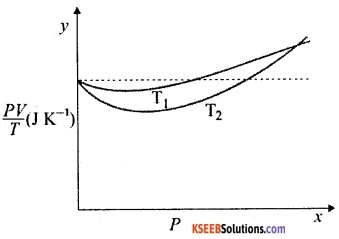1. The dotted plot signifies the behavior of equal amount of an ideal gas. Since the ideal gas satisfies the equation PV = nRT,
nR = $$\frac{P V}{T}$$ is a constant forgiven amount (n) of an ideal gas.
Thus $$\frac{P V}{T}$$ is independent of pressure.

2. The $$\frac{P V}{T}$$ curve at temperature T1 is closer to the dotted line (ideal gas) than the $$\frac{P V}{T}$$ curve at T2. Since a real gas at higher temperature behaves more like an ideal gas than a real gas at lower temperature, T1 >T2.

3. Since all three curves meet on the y-axis the value of $$\frac{P V}{T}$$ = nR, where n is the no of moles of ideal gas.
∴ n = no. of moles of oxygen gas
$$=\frac{\text { Given mass of oxygen }}{\text { gram molecular mass of oxygen }}$$
$$=\frac{1}{32}=0.31$$
∴ $$\frac{P V}{T}$$ = nR =0.031 × 8.314
= 0.26 J K-1

4. Since the molecular mass of hydrogen is less than that of oxygen, the number of moles is 1 g of hydrogen is more than that of oxygen. There fore, the value of $$\frac{P V}{T}$$ at the point where the curves meet the Y-axis will not be same for oxygen and hydrogen gases.
n = 0.031 mol of H2 will yield same $$\frac{P V}{T}$$
as that of 1 g of O2 gas.
∴ Mass of H2 gas
= 0. 311 × 2.02 = 0. 0626g

Question 4.
An oxygen cylinder of volume 30 liters has an initial gauge pressure of 15 atm and a temperature of 27 °C. After some oxygen is withdrawn from the cylinder, the gauge pressure drops to 11 atm and its temperature drops to 17 °C. Estimate the mass of oxygen taken out of the cylinder (R = 8.31 J mol-1 K-1, molecular mass of o2 = 32 u).
Initial Volume, V1 = 30 litres
= 30 × 10-3 m3
Initial Pressure P1 = 15 atm
= 15 × 101.3 KPa
Initial Tamperature, T1 = 27°C ≃ 300K
Let ‘n’ be the initial number of moles of oxygen.
From ideal gas law,
P1 V1 = n1 RT1
⇒ ∴ n1 = $$\frac{P_{1} V_{1}}{R T_{1}}$$
$$=\frac{\left(15.195 \times 10^{5}\right) \times 30 \times 10^{-3}}{8.314 \times 300}$$
= 18.276
Final volume, V2 = 30 litres = 30 × 10-3
Final pressure, P2 = 11 atm = 11.143 × 105
Final temperature, T2 = 17°C = 290 K
∴ Final no. of moles, n2 is given by.
n2 = $$\frac{P_{2} V_{2}}{R T_{2}}$$
$$=\frac{\left(11.143 \times 10^{5}\right) \times 30 \times 10^{-3}}{8.314 \times 290}$$
= 13.865
∴ Moles of oxygen taken out,
∆n = n1 – n2
= 18.276-13.865
= 4.411
∴ Mass of oxygen taken out
= ∆n × M
where M is the molecular mass of oxygen.
∴ mass of oxygen taken out = 4.411 × 32
= 141.152 g
∴ 141.152 g of oxygen was taken out of cylinder.Question 5.
An air bubble of volume 1.0 cm3 rises from the bottom of a lake 40 m deep at a temperature of 12 °C. To what volume does it grow when it reaches the surface, which is at a temperature of 35 ° C?
Initial volume of air bubble,
V1 = 1.0 cm3
= 10-6m3
Initial temperature, T1 = 12°C = 285K
Initial Pressure, P1= Ps + ρgh
where P1 = depth of air bubble = 40m
Ps = Pressure at lake surface
= 1atm
ρ = density of water = 102kg/cm3
g = acceleration due to gravity
= 9.8 ms-2
∴ P1 = 1.013 × 105+103 × 9.8 × 40
=  493300 Pa
=  4.933 × 105 Pa.
Let final volume of air bubble = V2
Final Pressure = Ps = 1.013 × 105 Pa
Final Temperature, T2 = 35°C = 308 K
From ideal gas law,
PV = n RT
∴ $$\frac{P V}{T}$$ = n R
∴ for given n, $$\frac{P V}{T}$$ = constant
⇒ $$\frac{P_{1} V_{1}}{T_{1}}=\frac{P_{2} V_{2}}{T_{2}}$$
∴ V2 = $$\frac{P_{1} V_{1} T_{2}}{T_{1} P_{2}}$$
$$=\frac{4.933 \times 10^{5} \times 10^{-6} \times 308}{285 \times 1.013 \times 10^{5}}$$
= 5.263 × 10-6 m3
= 5.263 cm3.
∴ The volume of air bubble grows to 5. 263 cm3 when it reaches the lake surface.

Question 6.
Estimate the total number of air molecules (Inclusive of oxygen, nitrogen, water vapour, and other constituents) in a room of capacity 25.0 m3at a temperature of 27 °C and 1 atm pressure.
From ideal gas law
PV = nRT
Here P = atm = 1. 013 × 105 Pa.
V = Volume = 25 m3
T = Temperature = 27°C = 300k
n = no of moles of gas
R = Gas constat = 8. 314 J mol-1 k-1
∴ n = $$\frac{P V}{R T}=\frac{1.013 \times 10^{5} \times 25}{8.314 \times 300}$$
= 0.01015 × 105 =1015 mol
Since 1 mol ≅ 6. 023 × 1023 molecules
N = 1015 mol ≅ 6.023 × 1023 × 1015
N = 6.113 × 1026 molecules.
∴ The total no of molecules in the given room is 6.113 × 1026.

Question 7.
Estimate the average thermal energy of a helium atom at
1. room temperature (27 °C),
2. the temperature on the surface of the Sun (6000 K),
3. the temperature of 10 million kelvin (the typical core temperature in the case of a star).
Average thermal energy of an atom
E= $$\frac{3}{2}$$ KT
where, K = Boltzmann constant
= 1.38 × 10-23m2kgs-2K-1
T = absolute temperature
1. room temperature (27°C)
T = 27°C = 300k
∴ E= $$\frac{3}{2}$$ × 1.38 × 10-23 × 300
= 1.242 × 10-21 J

2. Surface of the sun (6000 K)
T = 6000K
∴ E = $$\frac{3}{2}$$ × (1.38 × 10-23) × 6000
E = 1.242 × 10-19 J

3. core of a star (10 million K)
T = 10 × 106 = 107K
∴ E = $$\frac{3}{2}$$ × (1.38 × 10-23) × 107
E =2.07 × 10-16J.Question 8.
Three vessels of equal capacity have gases at the same temperature and pressure. The first vessel contains neon (monatomic), the second contains chlorine (diatomic), and the third contains uranium hexafluoride (polyatomic). Do the vessels contain equal number of respective molecules? Is the root mean square speed of molecules the same In the three cases? If not, in which case is Vrms the largest?
Since all vessels are of same capacity and are at same pressure and temperature,
P = P1 = P2 = P3 ……..(1)
V = V1 = V2 = V3 ……..(2)
T = T1 = T2 = T3 ……..(3)
where Pi Vi and Ti are pressure, volume and temperature of ith vessel respectively.
From ideal gas law
PV = nRT
∴ Pi Vi = ni RTi i = 1,2, 3
From equation (1), (2) and (3).
PV = ni RT
or ni = $$\frac{P V}{R T}$$ = constant for all the three vessels.
∴ All three vessels have same no of moles of respective gases. From Avagadro’s law, equal mols of gases contain equal no of molecules. The root mean square (RMS) speed of a molecule is given by
$$V_{r m s}=\sqrt{\frac{3 k_{B}}{m}}$$
where  kB = Boltzmann constant
T = temperature
m = molecular mass of the gas.
As Vrms α $$\frac{1}{\sqrt{\mathrm{m}}}$$ the rms speed of gas molecules in the three vessels are different. Since neon has the least molecular mass of the three gases, it has the highest rms speed.

Question 9.
At what temperature is the root mean square speed of an atom in an argon gas cylinder equal to the rms speed of a helium gas atom at – 20 ° C? (atomic mass of Ar= 39.9 u, of He = 4.0 u).
Temperature of helium gas,
T1= – 20°C = 253 k
gram molecular mass of Helium, m1 =4.0
gram molecular mass of Argon, m2 = 39.9 gm2
Let temperature of Argon = T2
The rms velocity of a gas atom is given by
$$\mathrm{V}_{\mathrm{rms}}=\sqrt{\frac{3 \mathrm{RT}}{\mathrm{M}}}$$
where T = Temperature of the gas
R = Universal Gas constant
M = Molar mass of the gas.
Given Vrms(Helium) = Vrms(Argon)
∴ $$\sqrt{\frac{3 \mathrm{RT}}{\mathrm{M}}}$$(Helium) = $$\sqrt{\frac{3 \mathrm{RT}}{\mathrm{M}}}$$(Argon)
$$\frac{T_{1}}{M_{1}}=\frac{T_{2}}{M_{2}}$$
∴ T2 = $$\frac{T_{1} M_{2}}{M_{1}}$$
$$=\frac{253 \times 39.9}{4}$$
= 2523.675 K
∴ At 2523.675 K, the rms speed of an atom of argon will be equal to that of helium at – 20°C.

Question 10.
Estimate the mean free path and collision frequency of a nitrogen molecule in a atm and temperature of 17 °C. Take the radius of a nitrogen molecule to be roughly 1.0 A. Compare the collision time with the time the molecule moves freely between two successive collisions (Molecular mass of N2 = 28.0 u).
The mean free path is given by
$$\ell=\frac{\mathrm{k} \mathrm{T}}{\sqrt{2} \pi \mathrm{d}^{2} \cdot \mathrm{P}}$$
where k = Bottzmann constant
= 1.38 × 10-23kg2m2s-2k-1
d = diameter of the atom / molecule
= 2 × 1 A° = 2 × 10-10 m
P = Pressure of the gas = 2 atm
= 2.026 × 105 Pa
$$\therefore \ell=\frac{1.38 \times 10^{-23} \times 290}{\sqrt{2} \times \pi \times\left(2 \times 10^{-10}\right)^{2} \times\left(2.026 \times 10^{5}\right)}$$
= 11.12 × 10-8 m
= 1.112 × 10-7 m
∴ mean free path = 1.112 × 10-7 m
RMS speed of nitrogen molecules is given by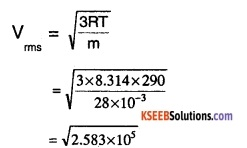Vrms = 508.26 ms-1
∴ collision frequency, f = $$\frac{V_{rms}}{\ell}$$
$$=\frac{508.26}{1.112 \times 10^{-7}}$$ = 4.57 × 109s-1
∴ collision time Tc = $$\frac{\mathrm{d}}{\mathrm{V}_{\mathrm{rms}}}$$
$$=\frac{2 \times 10^{-10}}{508.26}$$
= 3.935 × 10-13 s.
Time gap between successive collisions,
Tg = $$\frac{1}{f}$$
$$=\frac{1}{4.57 \times 10^{9}} \mathrm{s}$$
= 2.188 × 10-10s
$$\therefore \frac{T_{9}}{T_{c}}=\frac{2.188 \times 10^{-10}}{3.935 \times 10^{-13}}=555 \simeq 500$$
∴ the time taken between successive collisions is about 500 times the time spent on collision.

Question 11.
A metre long narrow bore held horizontally (and closed at one end) contains a 76 cm long mercury thread, which traps a 15 cm column of air. What happens if the tube Is held vertically with the open end at the bottom?
Total length of bore = 1 m = 100 cm.
length of air column = 15cm
length of mercury thread = 76cm.
∴ length of open space = 100 – (15 + 76)
= 9 cm
Initial pressure P1 = 76cm of mercury
Initial volume   V1= 15. A cm3
where, A = sectional area of the bore in cm2
Let h cm3 of mercury flow out of tube when held vertically
∴ New length of air column = 15 + 9 + h = 24 + h cm
New length of mercury = 76 – h cm
∴ Final pressure, P2 = 76 – (76-h)
= h cm of mercury
Find volume, V2 = (24 + h) × A cm3
Since temperature is constant and no air escapes the bore,
P1 V1 = P2 V2
∴ 76 × 15A = h (24+h) A
∴ 76 × 15 = h (24+h)
⇒ h = $$\frac{-24 \pm \sqrt{576+4560}}{2}$$
∴ h = $$\frac{-24 \pm 71.67}{2}$$
h = 23. 83, -47.83
Since height can only be positive, the amount of mercury that flows out of bore is 23. 83cm. There fore, mercury left in the bore = 52.2 cm and length of air column = 47.8 cm.Question 12.
From a certain apparatus, the diffusion rate of hydrogen has an average value of 28.7 cm3 s-1. The diffusion of another gas under the same conditions ie measured to have an average rate of 7.2 cm3 s-1. Identify the gas. [Hint: Use Graham’s law of diffusion: R1/R2 = ( M2/M1)1/2 , where R1, R2 are diffusion rates of gases 1 and 2, and M1 and M2 their respective molecular masses. The law is a simple consequence of kinetic theory.]
According to graham’s law of diffusion,
$$\frac{\mathrm{R}_{1}}{\mathrm{R}_{2}}=\left(\frac{\mathrm{M}_{1}}{\mathrm{M}_{2}}\right)^{1 / 2}$$
R1 = rate of diffusion of hydrogen
= 28.7cm3 S-1
R2 = Rate diffusion of unknown gas
= 7.2cm3 s-1
M1 = Molecular mass of hydrogen = 2.02
M2= Molecular mass of unknown gas.
∴ M2 = $$M_{1}\left(\frac{R_{1}}{R_{2}}\right)^{2}$$
= $$2.02\left(\frac{28.7}{7.2}\right)$$
= 32.09
The molecular mass of oxygen gas is 32. Hence, The given unknown gas is oxygen.

Question 13.
A gas in equilibrium has uniform density and pressure throughout its volume. This is strictly true only if there are no external influences. A gas column under gravity, for example, does not have uniform density (and pressure). As you might expect, its density decreases with height. The precise dependence is given by the so called law of atmospheres
n2 = n1 exp [ -mg (h2 – h1) /KBT] where n2, n1 refer to number density at heights h2 and h1 respectively. Use this relation to derive the equation for sedimentation equilibrium of a suspension in a liquid column:
n2 = n1 exp [-mg NA $$\left(\rho-p^{\prime}\right)$$ (h2-h1/ $$\boldsymbol{\rho}$$ RT)]
where ρ is the density of the suspended particle, and $$\rho^{\prime}$$ that of surrounding medium. [NA is Avogadro’s number, and R the universal gas constant.] [Hint: Use Archimedes principle to find the apparent weight of the suspended particle.]
From the law atmospheres , we have n2 = n1 exp (-mg (h2 -h1)/ KBT ]  …..(1)
where n2 = number density at height h2
n1 = number density at height h1
m = mass of the particle suspended in the column
Let
ρ = density of suspended particle
$$\rho^{\prime}$$ = density of the medium
m2 = mass of medium displaced
From Archimedes’ principle,
Effective weight of the suspended particle = Actual weight of the particle – weight of the medium displaced.
i.e., We = mg – $$m^{\prime}$$g
Let V be the volume displaced.
∴ $$\mathrm{m}^{1}=\mathrm{v}_{\mathrm{f}}^{\prime}$$ and $$\mathrm{V}=\left(\frac{\mathrm{m}}{\mathrm{f}}\right)$$
∴ $$\mathrm{m}^{\mathrm{l}}=\left(\frac{\mathrm{m}}{\rho}\right) \rho^{\mathrm{l}}$$
∴ effective weight, We = mg – m $$\left(\frac{\rho^{\prime}}{\rho}\right) \mathrm{g}$$
We = mg [1- $$\rho^{\prime}$$/ρ]   ……(2)
We know that
R = NA.kB (by definition)
where R = Universal Gas constant
kB = Boltzmann constant
∴ kB = R/NA …………(3)
Substituting the effective weight in (2) inplace of mg and using (3) in equation (1),
$$n_{2}=n_{1} \exp \left[m g\left(1-\frac{\rho^{1}}{\rho}\right)\left(h_{2}-h_{1}\right) /\left(R / N_{A}\right) T\right]$$
$$=n_{1} \exp \quad\left[\mathrm{mg} \mathrm{N}_{\mathrm{A}}\left(\rho-\rho^{\prime}\right)^{\left(\mathrm{h}_{2}-\mathrm{h}_{1}\right)} / \rho \mathrm{RT}\right]$$

Question 14.
Given below are densities of some solids and liquids. Give rough estimates of the size of their atoms :

 Substance Atomic mass(u) Density (103 kg nr3) Carbon (diamond) 12.01 2.22 Gold 197.00 19.32 Nitrogen (liquid) 14.01 1.00 Lithium 6.94 0.53 Fluorine (liquid) 19.00 1.!4

[Hint: Assume the atoms to be ‘tightly packed’ in a solid or liquid phase, and use the known value of Avogadro’s number. You should, however, not take the actual numbers you obtain for various atomic sizes too literally. Because of the crudeness of the tight packing approximation, the results only indicate that atomic sizes are in the range of a few A].
Assuming all molecules to be spherical, and solids and liquids are tightly packed,
Volume, V= $$\frac{4}{3} \pi r^{3}$$
where r = radius of a molecules
Also, V = $$\frac{\text { mass }}{\text { density }}$$
For 1 mole of a substance,
$$\mathrm{V}=\frac{4}{3} \pi \mathrm{r}^{3} \cdot \mathrm{N}_{\mathrm{A}}=\frac{\mathrm{M}}{\mathrm{J}}$$
where NA = Avogadro number = 6. 023 × 1023
M = gram atomic mass
ρ = density of the substute
∴ $$r=\left(\frac{3 M}{4 \pi \int N_{A}}\right)^{1 / 3}$$

1. Carbon (diamond):
M = 12.01g = 12.01 × 10-3Kg
f = 2.2 × 103 kgm-3
∴ $$r_{c}=\left(\frac{3 \times 12.01 \times 10^{-3}}{4 \pi \times 2.2 \times 10^{3} \times 6.023 \times 10^{23}}\right)$$
≃ 1.29 × 10-10m
rc ≃ 1.29 A°

2. Gold :
M = 197 g = 197 × 10-3 kg
f= 19.32 × 103 kg m-3
$$\therefore r_{9}=\left(\frac{3 \times 197 \times 10^{-3}}{4 \pi \times 19.32 \times 10^{3} \times 6.027 \times 10^{23}}\right)^{1 / 3}$$
= 1.59 × 10-10m
rg = 1.59 A°

3. Nitrogen (liquid):
M = 14.01g = 14.01 × 10-3kg
f = 103kgm-3
$$r_{n}=\left(\frac{3 \times 6.94 \times 10^{-3}}{4 \pi \times 0.53 \times 10^{3} \times 6.023 \times 10^{3}}\right)^{1 / 3}$$
= 1.73 A°

4. Fluorine (liquid):
M = 19 × 10-3kg
f = 1.14 × 103kgm-3
$$r_{n}=\left(\frac{3 \times 19 \times 10^{-3}}{4 \pi \times 1.14 \times 10^{3} \times 6.023 \times 10^{23}}\right)^{1 / 3}$$
= 1.876 A°

 Substance Radius Carbon (diamond) (A°) 1.29 Gold 1.59 Nitrogen (liquid) 1.77 Lithium 1.73 Fluorine (liquid) 1.88

### 1st PUC Physics Kinetic Theory One Mark Questions and Answers

Question 1.
Define mean free path.
The average distance covered by a moving molecule between successive collisions is called the mean free path.

Question 2.
Avagadro’s hypothesis states that under fixed conditions of temperature and pressure, equal volumes of all gases contain equal number of molecules.Question 3.
State Boyle’s law.
Boyle’s law states that under constant temperature, the pressure of given mass of a gas is inversely proportional to its volume.

Question 4.
State Charle’s low.
Charle’s law states that under constant pressure, the volume of a given mass of any gas is directly proportional to its temperature.

Question 5.
State law of equipartition of energy.
The law of equipartition of energy states that in thermal equilibrium, the total energy of a particle is equally distributed in all possible modes of energy, with each mode having an average energy equal to
$$\frac{1}{2}$$ kB T.

Question 6.
Avogadro number, NA, is defined as the number of molecules contained in 22.4 litres of any gas at STP, Its value is equal to 6.02 × 1023.

Question 7.
Calculate the number of molecules contained in 448 ml of an ideal gas at STP.
22.4 L contains 6.02 × 1023 molecules therefore 448 ml contains
N = $$\frac{448}{22400} \times 6.02 \times 10^{23}$$
∴ N = 12.04 × 1021
= 1.2 × 1022
∴ 448 ml of ideal gas at STP contains 1.2 × 1022molecules.Question 8.
If the temperature (absolute scale) of an ideal gas is doubled keeping pressure and volume constant, find the increase in its rms speed.
For an ideal gas,
$$\mathrm{V}_{\mathrm{ms}} \alpha \sqrt{\mathrm{T}}$$
There fore, if the temperature is doubled, the rms speed increases by a factor of $$\sqrt{2}$$.

### 1st PUC Physics Kinetic Theory Two Or Three Marks Questions and Answers

Question 1.
What is an ideal gas? Mention the ideal gas equation.
An ideal gas is a gas that obeys all the gas laws at all temperatures and is given by
PV = µRT
where P = pressure of the gas
V = Volume of the gas
µ = number of mols of the gas
R = Universal gas constant
T = absolute temperature .

Question 2.
A sample of gas is at 300K. Find the temperature at which the r.m.s speed of its molecules is doubled without changing the pressure.
Initial temperature, Ti = 300k
let final temperature, Tf = T
let initial rms speed, Vi = v1
∴ final rms speed, Vf = 2V1
For a gas of mass m and temperature T, the rms speed is given by
$$V_{\mathrm{rms}}=\sqrt{\frac{3 \mathrm{k}_{\mathrm{B}} \mathrm{T}}{\mathrm{m}}}$$
where kB = Boltzman constant.
∴ $$\frac{2 V_{1}}{V_{1}}=\sqrt{\frac{T}{300}}$$
∴ T = 300× (2)2
∴ T = 1200 k.

Question 3.
The temperature of an ideal gas is increasing from 100K to 500K if the rms speed of molecules at 100k Is V, what Is the rms speed of the molecules at 500K?
Initial temperature, Ti = 100K
final temperature of the gas Tf = 500K
Initial rms speed of molecules, Vrms,i = V
let final r.m.s speed of molecules Vrms,f = V’
for ideal gas,
$$V_{\mathrm{rms}}=\sqrt{\frac{3 \mathrm{k}_{\mathrm{B}} \mathrm{T}}{\mathrm{m}}}$$
where kB = Boltzmann constant
T = absolute temperature
m = mass of the gas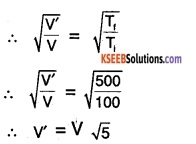There fore, the r.m.s speed of molecules of the given ideal gas at 500k is $$\sqrt{5}$$ V.Question 4.
At a given temperature T, the rms speed of Hydrogen molecules is V. Find rms speed of oxygen molecules at the same temperature.
Molecular mass of Hydrogen, MH2= 2
Molecular mass of Oxygen, MO2 = 32
rms speed of hydrogen gas VH2 = V
let rms speed of Oxygen gas, Vo2 = v’
The rms speed of gas is given by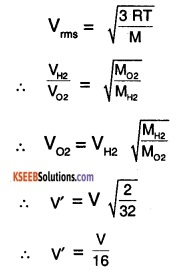∴ rms speed of oxygen at temperature T is $$\frac{\mathrm{V}}{16}$$.

Question 5.
A box contains N molecules of an ideal gas at temperature T and pressure P. The number of molecules in the box is increased to two times the original value. Find the final temperature and pressure if the kinetic energy of the gas is kept constant.
Initial no. of molecules ni = N
Initial temperature, Ti = T
Initial Pressure, Pi = P
Final no. of molecules, nf= 2N
Let final temperature, Tf=T’
let final pressure, Pf = p’
For ideal gases,
PV = $$\frac{2}{3}$$E ……..(1)
where P = pressure of the gas
V = volume of the gas
E = kinetic energy of molecules of the gas
Since E is constant, P is also a constant for the given case
∴ P’ = p
For ‘n’ mols of an ideal gas of molecular mass M,
$$\frac{1}{2} \mathrm{nM} \mathrm{V}_{\mathrm{rms}}^{2}=\frac{3}{2} \mathrm{nRT}$$
∴ E = $$\frac{3}{2}$$ NKBT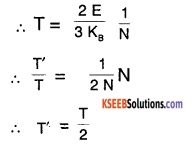Question 6.
Estimate the fraction of molecular volume to the actual volume occupied by Nitrogen gas at STP. Assume the radius of N2 = 300 pm.
Consider one mole of N2 gas at STP
∴ Actual volume, vact = 22.4L
vact = 22.4 × 10-3m3
molecular volume, Vmol= NA × $$\frac{4}{3}$$ × π ×R3
∴ Vmol = 6.81 × 10-5m3
∴ Ratio of molecular volume to actual volume,
$$\frac{V_{\text {mol }}}{V_{\text {act }}}=\frac{6.81 \times 10^{-5}}{22.4 \times 10^{-3}}$$
= 3.04 × 1 O-3

Question 7.
The molecules of a given mass of an ideal gas have rms speed 100 ms-1at 273K and 100kPa calculate the rms speed of the molecules if the temperature is doubled and pressure heated.
Initial temperature of gas, Ti = 273K
Final temperature of gas, Tf = 2 × Ti
= 546K
Initial rms speed of gas
molecules, Vi = 100 m/s
let final rms speed of molecules, Vf = V
For an ideal gas,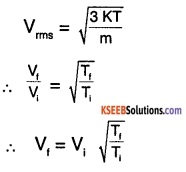∴ V = 100 $$\sqrt{2}$$
∴ V = 141.4 m/s.

Question 8.
State and explain Boyle’s law.
Boyle’s law states that at constant temperature, the volume of a given mass of an ideal gas is inversely proportional to its pressure.
Let the volume of the gas be ‘V’ and pressure ‘P’ and temperature, T. Then according to Boyle’s law,
$$\mathrm{V} \propto \frac{1}{\mathrm{P}}$$
or V =$$\frac{k}{P}$$ ⇒ V.P = k.
where k = proportionality constant i.e, according to Boyle’s law, if the temperature is kept constant, for a given change in volume the pressure changes such that the product of pressure and volume is a constant.Question 9.
State and explain Charles law.
Charles’ law states that at constant pressure, the volume of a given mass of an ideal gas is directly proportional to its temperature.
consider a gas at pressure ‘P’, temperature ‘T’ having volume ‘V’, Then Charles’ law states that
$$\mathrm{V} \propto \mathrm{T}$$
or V = kT where k is the proportionality constant.

Question 10.
At what temperature the molecules of nitrogen will have the same rms velocity as the molecules of oxygen at 400K?
Molecular mass of Nitrogen, M1 =28
Molecular mass of Oxygen, M2 = 32
Temperature of the Oxygen, T2 = 400
let temperature of nitrogen, T1= T
The rms speed of gas molecules given by,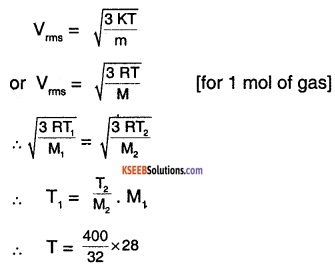∴ T = 350 K

### 1st PUC Physics Kinetic Theory Four/Five Marks Questions and Answers

Question 1.
Derive the ratio of specific heats for a mono atomic and diatomic gases
1. Monatomic gas :
No of degrees of freedom = 3 (Translational) of each molecule
∴ Average kinetic energy of a molecule at temperature T, E = 3 $$\frac{1}{2}$$ × kBT
where kB = Boltzmonn constant
U = $$\frac{3}{2}$$ kBT. NA [Total internal energy of the gas]
∴ U = $$\frac{3}{2}$$ R T
The molar specific heat at constant volume,
Cv = $$\frac{\mathrm{d} \mathrm{U}}{\mathrm{d} \mathrm{T}}$$
= $$=\frac{d}{d T}\left(\frac{3}{2} R T\right)=\frac{3}{2} R$$ ……..(1)
For ant ideal gas
Cp – Cv = R …………(2)
Using equation (1) in equation (2),
Cp = R + $$\frac{3}{2}$$ R = $$\frac{5}{2}$$ R
∴ ratio of specific heats,
$$\gamma=\frac{\mathrm{C}_{\mathrm{p}}}{\mathrm{W}}=\frac{\left(\frac{5}{2}\right) \mathrm{R}}{\left(\frac{3}{2}\right) \mathrm{R}}=\frac{5}{3}$$

2. Diatomic gas:
Case 1:
molecules are rigid
Number of degrees of freedom of each molecules = 5 (3 translational + 2 rotational)
∴ Average energy per molecule
= 5 × $$\frac{1}{2}$$ kBT = $$\frac{5}{2}$$ kBT
∴ Total internal energy
U = $$\frac{5}{2}$$ RT
Cv $$\frac{d}{d T}\left(\frac{5}{2} R T\right)$$
= $$\frac{5}{2}$$ R …….(3)
∴ Using equation (3) in equation (2),
Cp – $$\frac{5}{2}$$ R = R
∴ Ratio of specific heats for diatomic gas,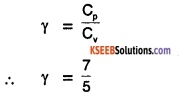case 2:
diatomic molecules are not rigid,
Average energy per molecule
= 5 × $$\frac{1}{2}$$ kBT + kBT =$$\frac{7}{2}$$ kBT
∴ Internal energy of the gas, U = $$\frac{7}{2}$$ RT
$$C_{v}=\frac{d}{d T}\left(\frac{7}{2} R T\right)=\frac{7}{2} R$$ ……(4)
Using equation (4) in equation (2),
Cp = $$\frac{9}{2}$$ R
∴ Ratio of specific heats,
γ = $$\frac{9}{7}$$.